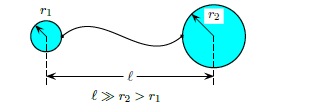# Problem: Two charged spherical conductors are connected by a long conducting wire. A total charge of q &gt; 0 is placed on this combination of two spheres. Sphere 1 has a radius of r1 and sphere 2 has a radius of r2, where r2 &gt; r1. If q1 represents the charge on sphere 1 and q 2 the charge on sphere 2, what is the ratio q1 / q2 of the charges? 1. q1 / q2 = r2 / r1 2. q1 / q2 = r1 / r1 + r2 3. q1 / q2 = (r2 / r1)2 4. q1 / q2 = r2 / r1 + r2 5. None of these 6. q1 / q2 = 1  7. q1 / q2 = (r1 / r2)2 8. q1 / q2 = r1 / r2

🤓 Based on our data, we think this question is relevant for Professor Heidarian's class at UMD.

###### Problem Details

Two charged spherical conductors are connected by a long conducting wire. A total charge of q > 0 is placed on this combination of two spheres. Sphere 1 has a radius of r1 and sphere 2 has a radius of r2, where r2 > r1. If q1 represents the charge on sphere 1 and q 2 the charge on sphere 2, what is the ratio q1 / q2 of the charges?

1. q1 / q2 = r2 / r1

2. q1 / q2 = r1 / r1 + r2

3. q1 / q2 = (r2 / r1)2

4. q1 / q2 = r2 / r1 + r2

5. None of these

6. q1 / q2 = 1

7. q1 / q2 = (r1 / r2)2

8. q1 / q2 = r1 / r2